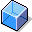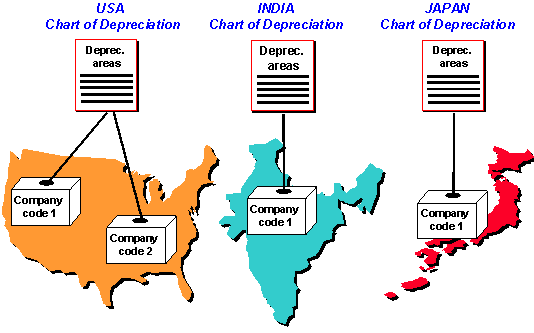Chart of DepreciationDefinition

Charts of depreciation are used in order to manage various legal requirements for the depreciation and valuation of assets. These charts of depreciation are usually country-specific and are defined independently of the other organizational units. A chart of depreciation, for example, can be used for all the company codes in a given country (refer to Company Code Assignment ).Country-Specific Charts of Depreciation

In the simplest scenario, all of your company codes are in the same country and are subject to the same legal requirements for asset valuation, meaning that you only need one chart of depreciation.

Structure

The chart of depreciation consists of the following parts:

• In general, you are required to calculate values for assets for different needs, both internal and external (such as book depreciation and cost depreciation). Therefore, the Asset Accounting component enables you to manage values for assets in parallel in up to 99 depreciation areas. The chart of depreciation, therefore, is a directory of depreciation areas organized according to business management requirements. You define the characteristics, and thereby the significance, of the individual depreciation areas in each chart of depreciation. A depreciation area is always assigned to only one chart of depreciation.
• You flexibly define the keys for the automatic depreciation of assets in each chart of depreciation. They are based on elements for calculation (calculation methods, period controls, and so on) that are available client-wide.

You can change and add to the standard calculation keys that are delivered with the system (refer to Depreciation )

• There are specific objects in the chart of depreciation for special calculations of asset values (for example, investment support keys for investment support - refer to Special Valuation ).

Use

SAP supplies typical reference charts of depreciation for each country. They have different depreciation areas and depreciation keys depending on that country’s specific requirements. You cannot use these charts of depreciation directly. You must create your own chart of depreciation by copying the reference chart of depreciation. Delete any depreciation areas that are not needed.

You can document the meaning of any chart of depreciation you set up in the system by writing a description for it.The graphic below shows the standard chart of depreciation for the USA.Integration

Company Code/Chart of Depreciation

You have to assign each company code defined in Asset Accounting to exactly one chart of depreciation. In the interests of keeping asset values uniform in your company, you should restrict the number of charts of depreciation used to as few as possible. Company codes in countries with the same valuation rules or company codes of a certain industry sector generally use the same chart of depreciation.

Chart of Accounts/Chart of Depreciation

The assignment of a company code to a chart of accounts is independent from its assignment to a chart of depreciation. This means that several company codes can use the same chart of accounts, although they have different charts of depreciation (and vice versa).

Refer to: